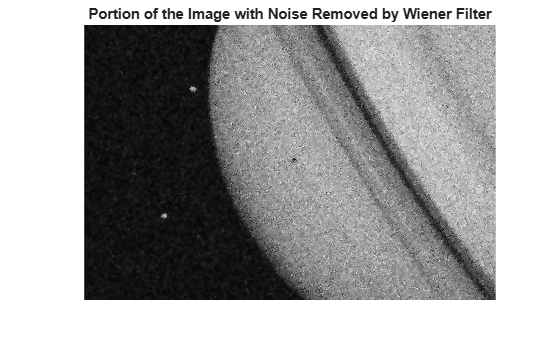## Noise Removal

Digital images are prone to various types of noise. Noise is the result of errors in the image acquisition process that result in pixel values that do not reflect the true intensities of the real scene. There are several ways that noise can be introduced into an image, depending on how the image is created. For example:

• If the image is scanned from a photograph made on film, the film grain is a source of noise. Noise can also be the result of damage to the film, or be introduced by the scanner itself.

• If the image is acquired directly in a digital format, the mechanism for gathering the data (such as a CCD detector) can introduce noise.

• Electronic transmission of image data can introduce noise.

To simulate the effects of some of the problems listed above, the toolbox provides the `imnoise` function, which you can use to add various types of noise to an image. The examples in this section use this function.

### Remove Noise by Linear Filtering

You can use linear filtering to remove certain types of noise. Certain filters, such as averaging or Gaussian filters, are appropriate for this purpose. For example, an averaging filter is useful for removing grain noise from a photograph. Because each pixel gets set to the average of the pixels in its neighborhood, local variations caused by grain are reduced.

See What Is Image Filtering in the Spatial Domain? for more information about linear filtering using `imfilter`.

### Remove Noise Using an Averaging Filter and a Median Filter

This example shows how to remove salt and pepper noise from an image using an averaging filter and a median filter to allow comparison of the results. These two types of filtering both set the value of the output pixel to the average of the pixel values in the neighborhood around the corresponding input pixel. However, with median filtering, the value of an output pixel is determined by the median of the neighborhood pixels, rather than the mean. The median is much less sensitive than the mean to extreme values (called outliers). Median filtering is therefore better able to remove these outliers without reducing the sharpness of the image.

Note: Median filtering is a specific case of order-statistic filtering, also known as rank filtering. For information about order-statistic filtering, see the reference page for the `ordfilt2` function.

Read image into the workspace and display it.

```I = imread('eight.tif'); figure imshow(I)```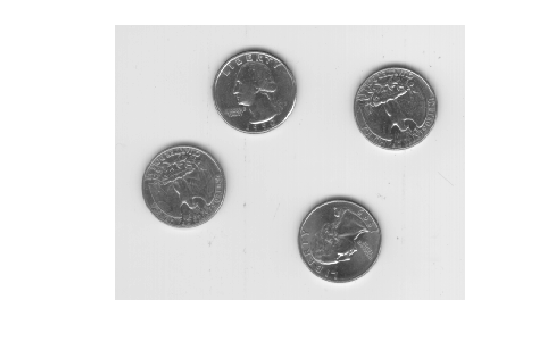For this example, add salt and pepper noise to the image. This type of noise consists of random pixels being set to black or white (the extremes of the data range).

```J = imnoise(I,'salt & pepper',0.02); figure imshow(J)```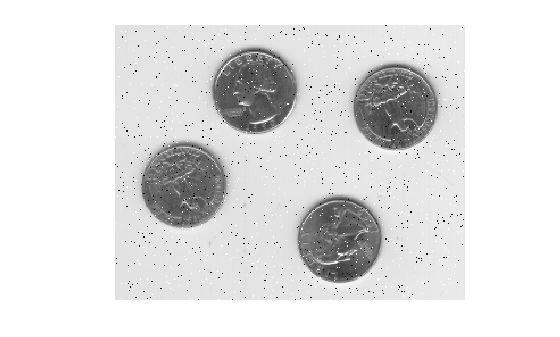Filter the noisy image, `J`, with an averaging filter and display the results. The example uses a 3-by-3 neighborhood.

```Kaverage = filter2(fspecial('average',3),J)/255; figure imshow(Kaverage)```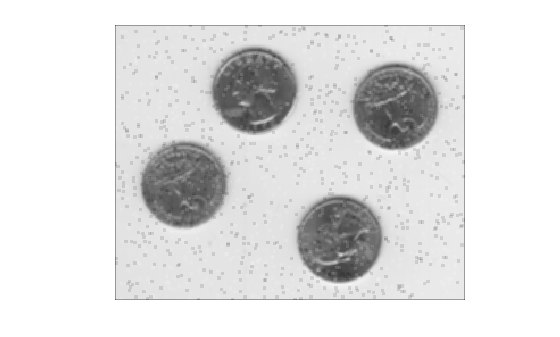Now use a median filter to filter the noisy image, `J`. The example also uses a 3-by-3 neighborhood. Display the two filtered images side-by-side for comparison. Notice that `medfilt2` does a better job of removing noise, with less blurring of edges of the coins.

```Kmedian = medfilt2(J); imshowpair(Kaverage,Kmedian,'montage')```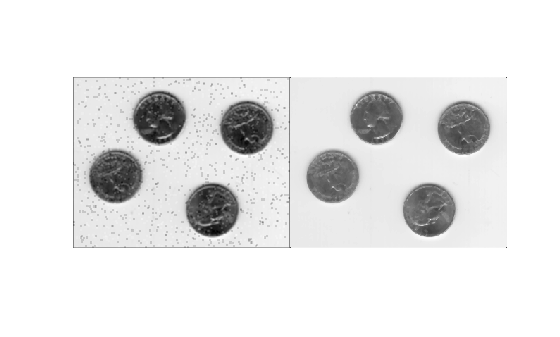### Remove Noise By Adaptive Filtering

This example shows how to use the `wiener2` function to apply a Wiener filter (a type of linear filter) to an image adaptively. The Wiener filter tailors itself to the local image variance. Where the variance is large, `wiener2` performs little smoothing. Where the variance is small, `wiener2` performs more smoothing.

This approach often produces better results than linear filtering. The adaptive filter is more selective than a comparable linear filter, preserving edges and other high-frequency parts of an image. In addition, there are no design tasks; the `wiener2` function handles all preliminary computations and implements the filter for an input image. `wiener2`, however, does require more computation time than linear filtering.

`wiener2` works best when the noise is constant-power ("white") additive noise, such as Gaussian noise. The example below applies `wiener2` to an image of Saturn with added Gaussian noise.

Read the image into the workspace.

`RGB = imread('saturn.png');`

Convert the image from truecolor to grayscale.

`I = rgb2gray(RGB);`

Add Gaussian noise to the image

`J = imnoise(I,'gaussian',0,0.025);`

Display the noisy image. Because the image is quite large, display only a portion of the image.

```imshow(J(600:1000,1:600)); title('Portion of the Image with Added Gaussian Noise');```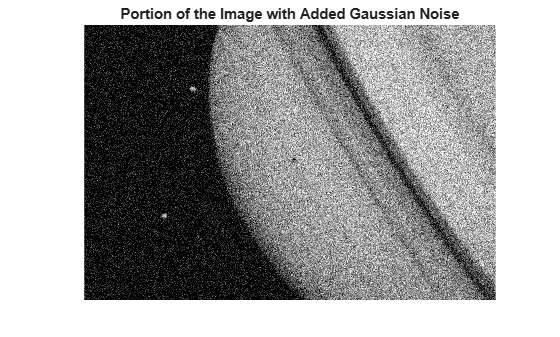Remove the noise using the `wiener2` function.

`K = wiener2(J,[5 5]);`

Display the processed image. Because the image is quite large, display only a portion of the image.

```figure imshow(K(600:1000,1:600)); title('Portion of the Image with Noise Removed by Wiener Filter');```